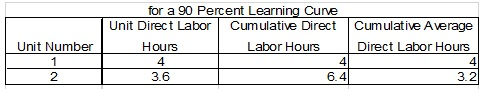# How is this calculated Hourly rate = \$22 Rate per repair = \$88 (\$22 per hour x 4 hours) Learning rat

How is this calculated Hourly rate = \$22 Rate per repair = \$88 (\$22 per hour x 4 hours) Learning rate = 90% Dealers recommendation: Average time for the 1st unit repaired = 9.6 hours Average time for the 2nd unit repaired = 7.2 hours Suggested rate per repair = \$110 Preliminary solutions addressing questions raised in item no.1 and taking into account observations listed in item no. 2: Arithmetic Procedure: Logarithmic Procedure: Y2 = (4) (2) log 0.9/log 2 = (4) (2) -0.322 = 4/(2) 0.322 = 4/1.25 = 3.2 Therefore: three repairs in an hour – I do not understand where the 1.25 is coming from and I can not reproduce this calculation to get the 3.6 (I get 3.7) also I do not understand how the 6.4 was calculated. Appreciate any help!for a 90 Percent Learning Curve Unit Direct Labor Cumulative Direct Cumulative Average Direct Labor Hours Unit Number Hours 4 3.6 Labor Hours 4 6.4 4 3.2 2